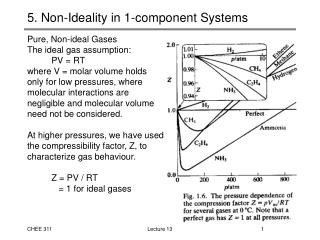Download Presentation5. Non-Ideality in 1-component Systems

# 5. Non-Ideality in 1-component Systems - PowerPoint PPT PresentationDownload Presentation## 5. Non-Ideality in 1-component Systems

- - - - - - - - - - - - - - - - - - - - - - - - - - - E N D - - - - - - - - - - - - - - - - - - - - - - - - - - -
##### Presentation Transcript

1. 5. Non-Ideality in 1-component Systems • Pure, Non-ideal Gases • The ideal gas assumption: • PV = RT • where V = molar volume holds • only for low pressures, where • molecular interactions are • negligible and molecular volume • need not be considered. • At higher pressures, we have used • the compressibility factor, Z, to • characterize gas behaviour. • Z = PV / RT • = 1 for ideal gases Lecture 13

2. Gibbs Energy of Pure Gases • For any pure gas, ideal or non-ideal, the fundamental equation applies: • dG = VdP - SdT • At constant T, changes in the Gibbs energy of a pure gas arise only from changes in pressure, and: • dG = VdP (constant T) • We can integrate between two pressures, Pref and P to obtain: • For an ideal gas, we can substitute for the molar volume, V=RT/P Lecture 13

3. Gibbs Energy of Pure, Ideal Gases • For the ideal gas case, we have • If we consistently select unit pressure (1 bar, 1 psi, etc) as our reference state, we can simplify the expression: • 11.28 • where i(T) is only a function of temperature. • This expression provides the Gibbs energy per mole of a pure, ideal gas at a given P and T • We would like to develop an analogous expression for non-ideal systems, for which V RT/P • Like all non-ideal systems, we can’t predict how V,T and P relate, but we can perform experiments and correlate our data Lecture 13

4. Gibbs Energy for Pure, Non-ideal Gases • The utility of Equation 11.28 leads us to define a direct analogue • 11.31 • where • i(T) the same function of temperature • fi is a defined intensive variable called the fugacity (units of pressure) • Fugacity is used to describe the Gibbs energy of non-ideal gases. In these cases, Gibbs energy does not vary with ln(P), so we define a new “chemical pressure” such that the Gibbs energy varies directly with ln fi. • Equation 11.31 is the first part of the definition of fugacity. The second part specifies that as the pressure approaches zero (and the pure gas becomes more ideal) the fugacity approaches the pressure. Lecture 13

5. Pure Gases: Fugacity and Fugacity Coefficient • In summary, the fugacity of a pure, non-ideal gas is defined as: • with the specification that: • Together, these definitions allow us to quantify the Gibbs energy of non-ideal gases. • A closely related parameter is the fugacity coefficient, defined by: • such that • Note that a gas behaving ideally is defined as having fi = 1, in which case the expression reduces to equation 11.28. Lecture 13

6. Calculating the Fugacity of a Pure Gas • The simplest means of calculating the fugacity of a pure gas is to compare its behaviour to an ideal system. We will do this frequently in our treatment of non-ideality. • For the non-ideal gas: • For the ideal gas: • Taking the difference of these equations: Lecture 13

7. Calculating the Fugacity of a Pure Gas • We can simplify this relation by an appropriate choice of Pref. As pressure goes to zero, a real gas approaches ideality. Therefore, • With Pref = 0, we have: • or • Substituting V = ZRT/P and Vig = RT/P, we arrive at: • 11.35 Lecture 13

8. Calculating the Fugacity of a Pure Gas • Equation 11.35 is commonly written in terms of the fugacity coefficient: • at a given T. • To calculate the fugacity of a pure, non-ideal gas, all we need is information on the relationship of Z as a function of P at T. • Experimental data • Equations of State • Generalized correlations Lecture 13